# Local Outlier Detection with ssMRCD

We use this vignette to reproduce the real world example for local outlier detection analysed and described in Puchhammer and Filzmoser (2023). All functions are included in the package ssMRCD.

library(ssMRCD)
library(ggplot2)

## Data Preparation

The original data from GeoSphere Austria (2022) is pre-cleaned and saved in the data frame object weatherAUt2021. Additional information can be found on the helping page.

? weatherAUT2021
data("weatherAUT2021")
#>        p     s   vv    t rsum  rel             name      lon      lat  alt
#> 1 941.35 154.5 2.15 6.15 49.5 77.5    AIGEN/ENNSTAL 14.13833 47.53278  641
#> 2 945.80 172.5 2.95 6.25 39.5 76.0      ALLENTSTEIG 15.36694 48.69083  599
#> 3 985.50 152.0 2.55 8.20 46.5 78.0        AMSTETTEN 14.89500 48.10889  266
#> 4 893.20 123.5 1.85 5.10 66.0 74.5      BAD GASTEIN 13.13333 47.11055 1092
#> 5 984.50 176.0 1.50 8.45 40.5 75.0 BAD GLEICHENBERG 15.90361 46.87222  269
#> 6 992.05 188.5 2.20 8.95 60.5 77.0  BAD RADKERSBURG 15.99333 46.69222  207

To apply the local outlier detection function local_outliers_ssMRCD we need to specify a structure to calculate the ssMRCD estimator, meaning we need a neighborhood assignment and a weight matrix specifying the relative influence of the neighborhoods on each.

Since a prominent part of Austria is Alpine landscape small sized neighborhoods might be appropriate. Thus, we construct a grid of the longitude and latitude values and classify the observations. We summarize neighborhoods for a very small number of observations.

cut_lon = c(9:16, 18)
cut_lat = c(46, 47, 47.5, 48, 49)
N = ssMRCD::N_structure_gridbased(weatherAUT2021$lon, weatherAUT2021$lat, cut_lon, cut_lat)
table(N)
#> N
#>  1  2  3  4  5  6  7  8  9 10 11 12 13 14 15 16 17 18 19 20 21 22 23 24 25 26
#>  6  1  1 12  1  1  9  5  9  3  1  8  6  7  8 12  6  5  9  5  7  7 17  7  9 21
N[N == 2] = 1
N[N == 3] = 4
N[N == 5] = 4
N[N == 6] = 7
N[N == 11] = 15
N = as.numeric(as.factor(N))

The final neighborhood structure can be seen in the following plot, where the neighborhoods are differently colorized.

g1 = ggplot() +
geom_text(data = weatherAUT2021, aes(x = lon, y = lat, col = as.factor(N), label = N)) +
geom_hline(aes(yintercept = cut_lat), lty = "dashed", col = "gray") +
geom_vline(aes(xintercept = cut_lon), lty = "dashed", col = "gray") +
labs(x = "Longitude", y = "Latitude", title = "Austrian Weather Stations: Neighborhood Structure") +
coord_fixed(1.4) +
theme_classic() +
theme(legend.position = "none")
g1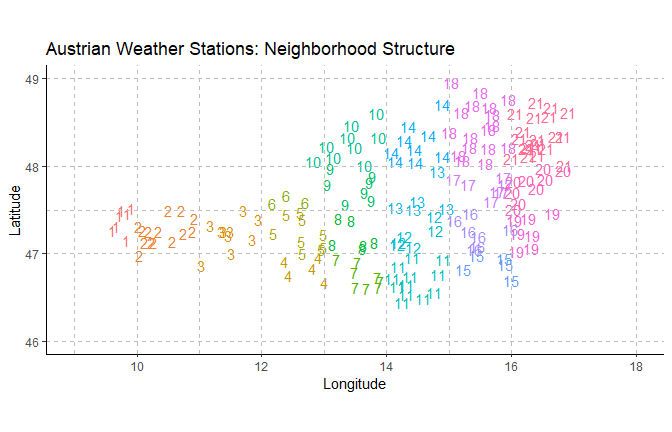A natural choice for the weight matrix when using a grid-based neighborhood structure is the geographical inverse-distance weighting matrix. It is the default value for the local_outliers_ssMRCD function but can explicitly be calculated using the function geo_weights. This function returns also the center of each neighborhood. E.g. for neighborhood 4 we have the following weights, corresponding to the transparancy of the arrows.

GW = geo_weights(coordinates = weatherAUT2021[, c("lon", "lat")],
N_assignments = N)
GW$W[4, ] #> 1 2 3 4 5 6 7 #> 0.02554129 0.03265041 0.06004253 0.00000000 0.19041215 0.09170249 0.08164324 #> 8 9 10 11 12 13 14 #> 0.08849001 0.06215254 0.04767836 0.04370851 0.04285327 0.03754845 0.03340820 #> 15 16 17 18 19 20 21 #> 0.02547782 0.02752795 0.02472584 0.02375660 0.02127099 0.02047626 0.01893310 g1 + labs(title = "Austrian Weather Stations: Weighting Matrix W") + geom_segment(aes(x = GW$centersN[4, 1],
y = GW$centersN[4, 2], xend = GW$centersN[-4, 1]-0.1,
yend = GW$centersN[-4, 2]-0.05, alpha = GW$W[4, -4]),
arrow = arrow(length = unit(0.25, "cm")),
linewidth = 2,
col = "blue")+
geom_text(aes(x = GW$centersN[, 1], y = GW$centersN[, 2], label = 1:length(GW$centersN[, 2])))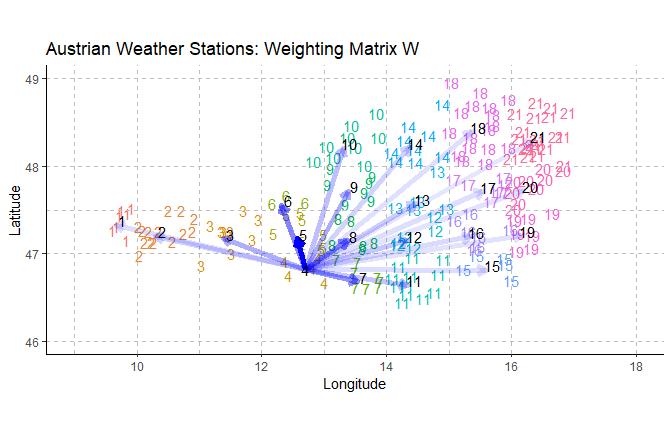## Parameter Tuning Regarding the parameter settings we have many possibilities. When it comes to the number of neighbors we want to compare each observations with there are different possibilities. Either by specifying a value for k like k = 10 which gives the number of observations to compare with or the value for dist (e.g. dist = 1.5) as the radius of a circle around the observation where all observations inside are compared to the initial observation. A default value for k that is used among various local outlier detection methods is 10. However, depending on the spatial structure of the data it makes sense to use other values as well. For the spatial smoothing parameter lambda the restriction is it has to be in the interval [0, 1). One can set the values according to some argumentation based on the spatial distribution but also on underlying structures. A value of 0.5 is the default value. A more sophisticated approach is to introduce outliers to the data set and examine how well the parameter setting is able to find those. The function parameter_tuning can be used to analyse different combinations of k (or dist) and lambda (see also help page). ? parameter_tuning Observations are switched and thus, this should introduce outliers that we know of. Nevertheless you should keep in mind that the observations are switched entirely randomly. If unlucky we might switch similar observations and thus, the measured performance of the detection method might suffer. However, since we want to compare the different parameter settings on the same contaminated data sets this should not affect the fine tuning itself. Moreover, we implicitly assume that the original data set has no outliers. This is in general not the case. Thus, the false-negative rate (FNR) is not the true FNR regarding all outliers. Nevertheless, the parameter tuning is a way to see the effects of the parameter setting on the FNR and the false-positive rate (FPR) which is represented by the total number of found outliers. set.seed(123) parameter_tuning(data = weatherAUT2021[, 1:6], coords = weatherAUT2021[, c("lon", "lat")], N_assignments = N, repetitions = 3, k = c(5, 10, 15), lambda = c(0, 0.25, 0.5, 0.75))$plot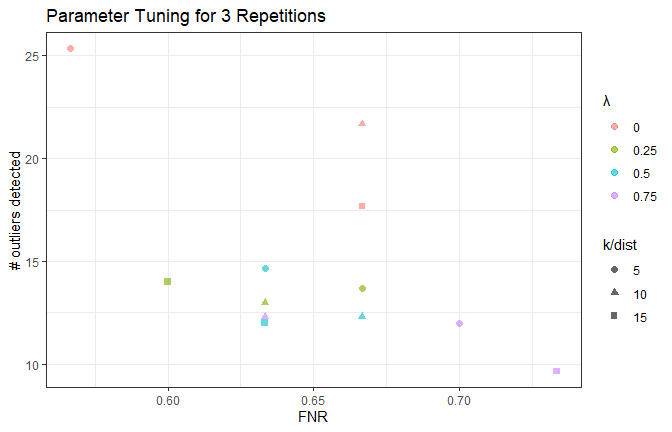It is apparent that some parameter settings are non-optimal compared to others. In general the final choice of the best parameter setting based on the output above depends on the overall goal. We choose lambda = 0.5 and k = 10. Although k = 10 is not the optimal choice here, the difference in performance is quite small and thus, we prefer to use the default value.

## Outlier Detection and Covariance Estimation

If we are only interested in the covariance estimation we can use the function ssMRCD. A more convenient way if we are interested in local outlier detection is to use the function local_outliers_ssMRCD, which already embeds the covariance estimation.

? local_outliers_ssMRCD
res = local_outliers_ssMRCD(data = weatherAUT2021[, 1:6],
coords = weatherAUT2021[, c("lon", "lat")],
N_assignments = N,
lambda = 0.5,
k = 10)
summary(res)
#> Local outliers: 10 of 183 observations (5.46%).
#> Used cut-off value: 14.20085.
#> Observations are compared to their k=10 neighbors.

The found outliers can be accessed by the list element outliers.

cat(weatherAUT2021$name[res$outliers], sep = ",\n")
#> OBERGURGL,
#> RUDOLFSHUETTE,
#> SCHOECKL,
#> PATSCHERKOFEL,
#> FEUERKOGEL,
#> LOIBL/TUNNEL,
#> SONNBLICK (TAWES),
#> RAX/SEILBAHNBERGSTATION,
#> GALZIG,
#> VILLACHER ALPE

### Diagnostics: Covariance Estimation

For the covariance estimation there are two diagnostic plots implemented for the S3-object "ssMRCD", which can be specified by type. For type "convergence" the convergence property for this specific data set is shown. For "ellipses"the estimated covariance matrices are shown in the first two eigenvectors fo the overall data set. The colour_scheme parameter yields even more insight into the covariances (see also ?plot.ssMRCD for more details).

covariance_res = res$ssMRCD plot(covariance_res, centersN = res$centersN,
manual_rescale = 0.5,
type = c("convergence", "ellipses"),
colour_scheme = "regularity",
legend = TRUE,
xlim = c(9, 19))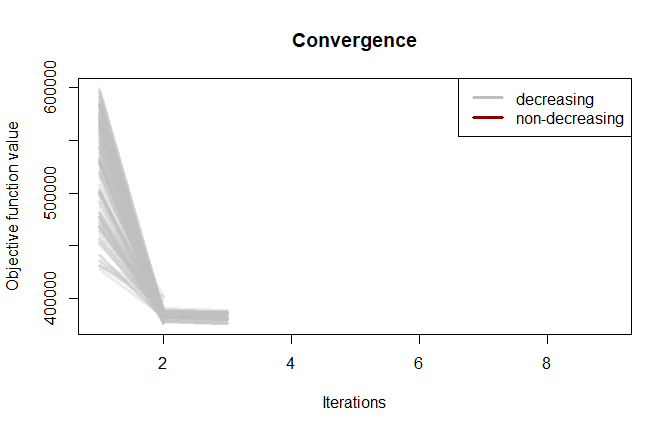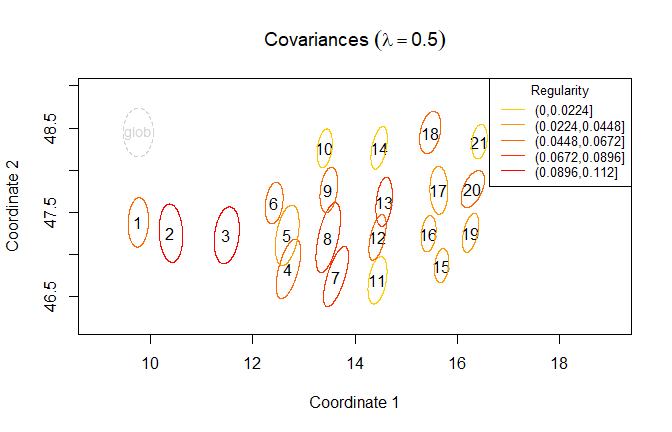For completeness the global biplot based on the global covariance matrix is also plotted:

biplot(stats:: princomp(weatherAUT2021[, 1:6],
cor  = TRUE,
covmat = robustbase::covMcd(weatherAUT2021[, 1:6])),
col = c("grey", "black"))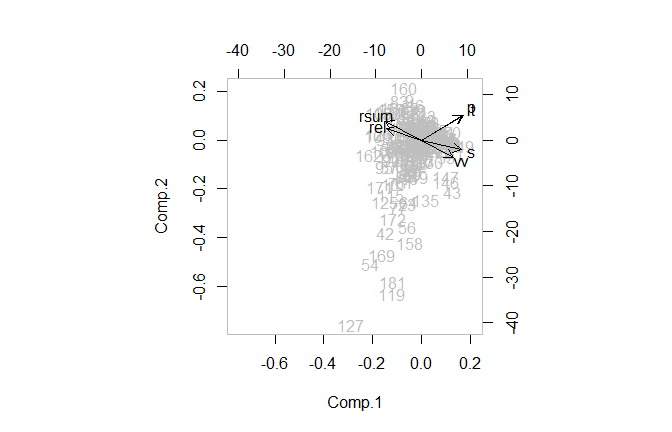### Diagnostics: Local Outlier Detection

For the local outlier detection method there are several plots regarding diagnostics available (see ?plot.locOuts). The histogram shows all next distances and the cut-off value. The spatial plot shows the outliers on the map, the 3D-plot as well, but with an additional axis for the next-distance/next-distance divided by cut-off value. The line plot shows the scaled values and the specified area in the map. Orange-coloured lines are other outliers on the map, the darkred colored line is the selected observation (focus).

? plot.locOuts
plot(res, type = "hist", pos = 4)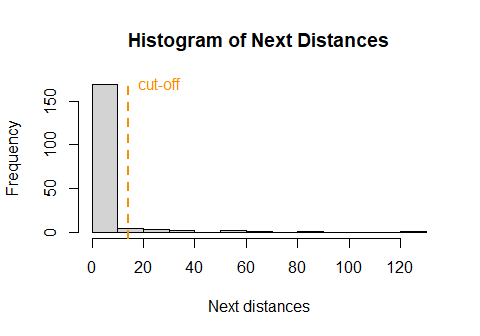plot(res, type = "spatial", colour = "outScore", xlim = c(9.5, 19))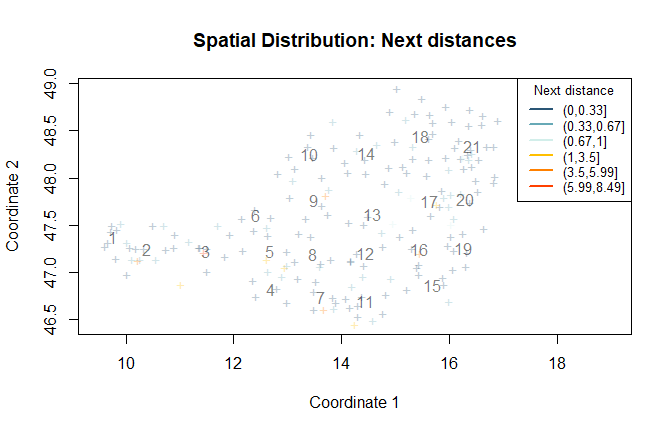# SCHOECKL
plot(res, type = "lines", focus = res\$outliers)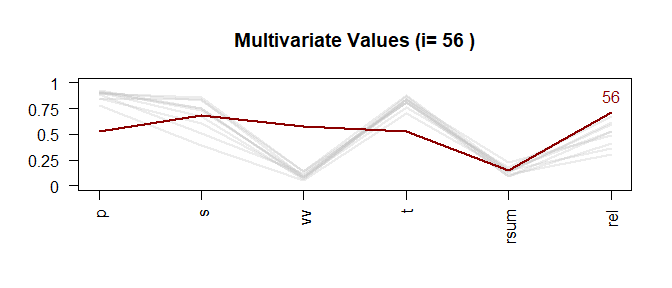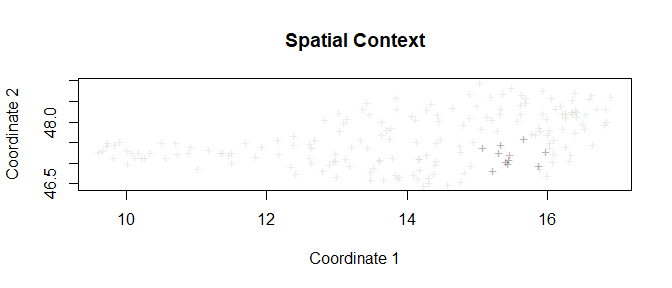plot(res, type = "3D", colour = "outScore", theta = 0, phi = 10)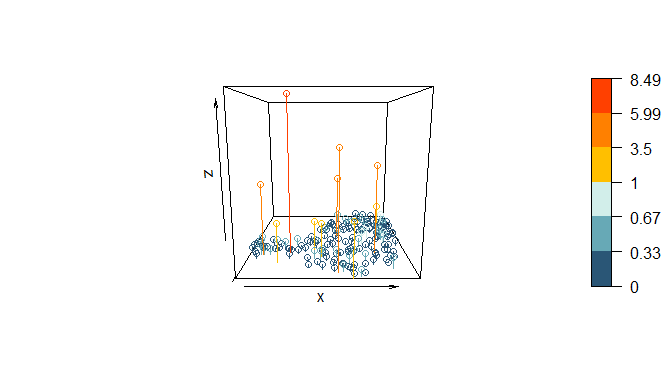If you want a fancy GIF you can also use the following code making use of the package animation. You should have ImageMagick installed.

library(animation)

# fineness of movement
n = 90
param = rbind(cbind(rep(0, 2*n + n/2), c(seq(90, 0, length.out = n), seq(0, 90, length.out = n), seq(90, 30, length.out = n/2))),
cbind(c(seq(0, 90, length.out = n), seq(90, 0, length.out = n)), rep(30, 2*n)),
cbind(rep(0, n/2), seq(30, 90, length.out = n/2)))

# use function saveGIF
saveGIF(interval = 0.2,
movie.name = "local_outliers_3D.gif",
expr = {
for (i in 1:dim(param)) {
plot(outs, type = "3D", colour = "outScore", theta = param[i, 1], phi = param[i, 2])
}}
)